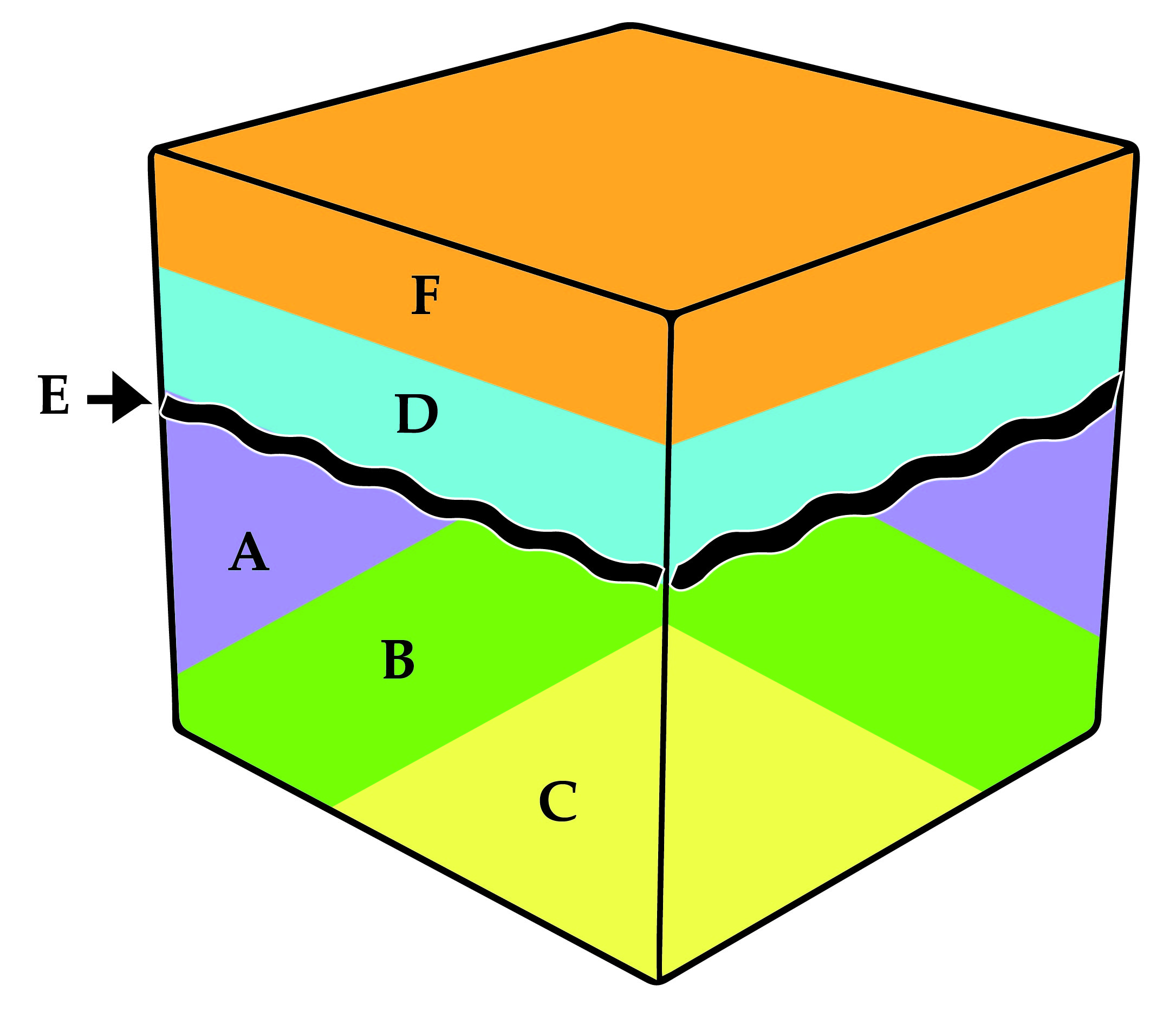# AP Environmental Science : Geological Time Scale

## Example Questions

### Example Question #71 : Ap Environmental Sciences

Geological time scales are used to measure spans of time. In terms of geological time scales, which category represents the smallest length of time?

Eon

Period

Supereon

Era

Period

Explanation:

The correct response is period. This would be the smallest measurement of time given the answer choices.

The order from longest to shortest is shown here: Supereon > Eon > Era > Period

### Example Question #1 : Soil And Geology

Geological time scales are used to measure spans of time. In terms of geological time scales, which category represents the longest length of time?

Age

Period

Epoch

Era

Era

Explanation:

The correct response is era because it represents longer periods of time on the geological time scale. The answer choicest from longest to shortest are as follows: Era > Period > Epoch > Age.

### Example Question #73 : Ap Environmental SciencesExamine the given block diagram. Each letter represents a depositional event or a period of erosion. Place the letters in order from the oldest to youngest event.

E, C, B, A, D, F

C, B, A, D, F, E

C, B, A, E, D, F

E, F, D, A, B, C

F, D, E, A, B, C